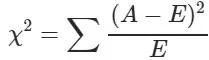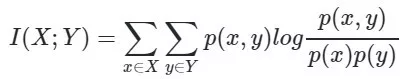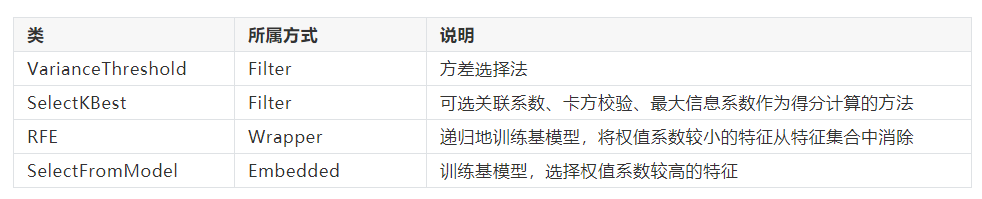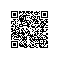# 为何推荐sklearn做单机特征工程？【下】

• 特征是否发散：如果一个特征不发散，例如方差接近于0，也就是说样本在这个特征上基本上没有差异，这个特征对于样本的区分并没有什么用。
• 特征与目标的相关性：这点比较显见，与目标相关性高的特征，应当优选选择。除方差法外，本文介绍的其他方法均从相关性考虑。

• Filter：过滤法，按照发散性或者相关性对各个特征进行评分，设定阈值或者待选择阈值的个数，选择特征。
• Wrapper：包装法，根据目标函数（通常是预测效果评分），每次选择若干特征，或者排除若干特征。
• Embedded：嵌入法，先使用某些机器学习的算法和模型进行训练，得到各个特征的权值系数，根据系数从大到小选择特征。类似于Filter方法，但是是通过训练来确定特征的优劣。

1. Filter

from sklearn.feature_selection import VarianceThreshold

#方差选择法，返回值为特征选择后的数据
#参数threshold为方差的阈值
VarianceThreshold(threshold=3).fit_transform(iris.data)

import numpy as np
from sklearn.feature_selection import SelectKBest
from scipy.stats import pearsonr

#选择K个最好的特征，返回选择特征后的数据
#第一个参数为计算评估特征是否好的函数，该函数输入特征矩阵和目标向量，输出二元组（评分，P值）的数组，数组第i项为第i个特征的评分和P值。在此定义为计算相关系数
#参数k为选择的特征个数
#SelectKBest(lambda X, Y: array(map(lambda x:pearsonr(x, Y), X.T)).T, k=2).fit_transform(iris.data, iris.target)

SelectKBest(lambda X,Y:np.array(list(map(lambda x:pearsonr(x, Y), X.T))).T, k=2).fit_transform(iris.data, iris.target)from sklearn.feature_selection import SelectKBest
from sklearn.feature_selection import chi2

#选择K个最好的特征，返回选择特征后的数据
SelectKBest(chi2, k=2).fit_transform(iris.data, iris.target)!pip install minepy
from sklearn.feature_selection import SelectKBest
from minepy import MINE

#由于MINE的设计不是函数式的，定义mic方法将其为函数式的，返回一个二元组，二元组的第2项设置成固定的P值0.5
def mic(x, y):
m = MINE()
m.compute_score(x, y)
return (m.mic(), 0.5)

#选择K个最好的特征，返回特征选择后的数据
#SelectKBest(lambda X, Y: array(map(lambda x:mic(x, Y), X.T)).T, k=2).fit_transform(iris.data, iris.target)
SelectKBest(lambda X, Y: array(list(map(lambda x:mic(x, Y), X.T))).T, k=2).fit_transform(iris.data, iris.target)

2.Wrapper

from sklearn.feature_selection import RFE
from sklearn.linear_model import LogisticRegression

#递归特征消除法，返回特征选择后的数据
#参数estimator为基模型
#参数n_features_to_select为选择的特征个数
RFE(estimator=LogisticRegression(), n_features_to_select=2).fit_transform(iris.data, iris.target)

3.Embedded

from sklearn.feature_selection import SelectFromModel
from sklearn.linear_model import LogisticRegression

#带L1惩罚项的逻辑回归作为基模型的特征选择
SelectFromModel(LogisticRegression(penalty="l1", C=0.1)).fit_transform(iris.data, iris.target)

L1惩罚项降维的原理在于保留多个对目标值具有同等相关性的特征中的一个，所以没选到的特征不代表不重要。故，可结合L2惩罚项来优化。具体操作为：若一个特征在L1中的权值为1，选择在L2中权值差别不大且在L1中权值为0的特征构成同类集合，将这一集合中的特征平分L1中的权值，故需要构建一个新的逻辑回归模型：

from sklearn.linear_model import LogisticRegression

class LR(LogisticRegression):
def __init__(self, threshold=0.01, dual=False, tol=1e-4, C=1.0,
fit_intercept=True, intercept_scaling=1, class_weight=None,
random_state=None, solver='liblinear', max_iter=100,
multi_class='ovr', verbose=0, warm_start=False, n_jobs=1):

#权值相近的阈值
self.threshold = threshold
LogisticRegression.__init__(self, penalty='l1', dual=dual, tol=tol, C=C,
fit_intercept=fit_intercept, intercept_scaling=intercept_scaling, class_weight=class_weight,
random_state=random_state, solver=solver, max_iter=max_iter,
multi_class=multi_class, verbose=verbose, warm_start=warm_start, n_jobs=n_jobs)
#使用同样的参数创建L2逻辑回归
self.l2 = LogisticRegression(penalty='l2', dual=dual, tol=tol, C=C, fit_intercept=fit_intercept, intercept_scaling=intercept_scaling, class_weight = class_weight, random_state=random_state, solver=solver, max_iter=max_iter, multi_class=multi_class, verbose=verbose, warm_start=warm_start, n_jobs=n_jobs)

def fit(self, X, y, sample_weight=None):
#训练L1逻辑回归
super(LR, self).fit(X, y, sample_weight=sample_weight)
self.coef_old_ = self.coef_.copy()
#训练L2逻辑回归
self.l2.fit(X, y, sample_weight=sample_weight)

cntOfRow, cntOfCol = self.coef_.shape
#权值系数矩阵的行数对应目标值的种类数目
for i in range(cntOfRow):
for j in range(cntOfCol):
coef = self.coef_[i][j]
#L1逻辑回归的权值系数不为0
if coef != 0:
idx = [j]
#对应在L2逻辑回归中的权值系数
coef1 = self.l2.coef_[i][j]
for k in range(cntOfCol):
coef2 = self.l2.coef_[i][k]
#在L2逻辑回归中，权值系数之差小于设定的阈值，且在L1中对应的权值为0
if abs(coef1-coef2) < self.threshold and j != k and self.coef_[i][k] == 0:
idx.append(k)
#计算这一类特征的权值系数均值
mean = coef / len(idx)
self.coef_[i][idx] = mean
return self

from sklearn.feature_selection import SelectFromModel

#带L1和L2惩罚项的逻辑回归作为基模型的特征选择
#参数threshold为权值系数之差的阈值
SelectFromModel(LR(threshold=0.5, C=0.1)).fit_transform(iris.data, iris.target)

from sklearn.feature_selection import SelectFromModel

#GBDT作为基模型的特征选择


4.回顾1. 主成分分析法（PCA）

from sklearn.decomposition import PCA

#主成分分析法，返回降维后的数据
#参数n_components为主成分数目
PCA(n_components=2).fit_transform(iris.data)

2. 线性判别分析法（LDA）

from sklearn.discriminant_analysis import LinearDiscriminantAnalysis as LDA

#线性判别分析法，返回降维后的数据
#参数n_components为降维后的维数
LDA(n_components=2).fit_transform(iris.data, iris.target)

⭐总结使用钉钉扫一扫加入圈子
+ 订阅